# Java 集合深入理解（16）：HashMap 主要特点和关键方法源码解读

## >点击查看 Java 集合框架深入理解 系列， - ( ゜- ゜)つロ 乾杯~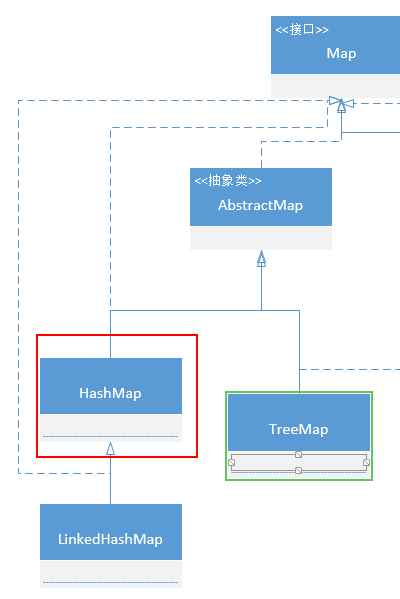## 什么是 HashMap

HashMap 是一个采用哈希表实现的键值对集合，继承自 AbstractMap，实现了 Map 接口
HashMap 的特殊存储结构使得在获取指定元素前需要经过哈希运算，得到目标元素在哈希表中的位置，然后再进行少量比较即可得到元素，这使得 HashMap 的查找效率贼高。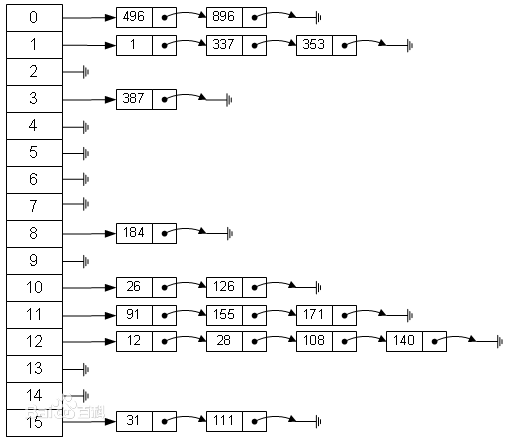## HashMap 的特点

• 底层实现是 链表数组，JDK 8 后又加了 红黑树
• 实现了 Map 全部的方法
• key 用 Set 存放，所以想做到 key 不允许重复，key 对应的类需要重写 hashCode 和 equals 方法
• 允许空键和空值（但空键只有一个，且放在第一位，下面会介绍）
• 元素是无序的，而且顺序会不定时改变
• 插入、获取的时间复杂度基本是 O(1)（前提是有适当的哈希函数，让元素分布在均匀的位置）
• 遍历整个 Map 需要的时间与 桶(数组) 的长度成正比（因此初始化时 HashMap 的容量不宜太大）
• 两个关键因子：初始容量、加载因子

## HashMap 的 13 个成员变量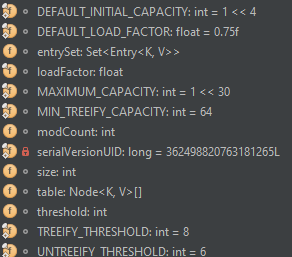1.默认初始容量：16，必须是 2 的整数次方

static final int DEFAULT_INITIAL_CAPACITY = 1 << 4;


2.默认加载因子的大小：0.75，可不是随便的，结合时间和空间效率考虑得到的

static final float DEFAULT_LOAD_FACTOR = 0.75f;


3.最大容量： 2^ 30 次方

static final int MAXIMUM_CAPACITY = 1 << 30;


4.当前 HashMap 修改的次数，这个变量用来保证 fail-fast 机制

transient int modCount;


5.阈值，下次需要扩容时的值，等于 容量*加载因子

int threshold;


6.树形阈值：JDK 1.8 新增的，当使用 树 而不是列表来作为桶时使用。必须必 2 大

static final int TREEIFY_THRESHOLD = 8;


7.非树形阈值：也是 1.8 新增的，扩容时分裂一个树形桶的阈值（？不是很懂 - -），要比 TREEIFY_THRESHOLD 小

static final int UNTREEIFY_THRESHOLD = 6;


8.树形最小容量：桶可能是树的哈希表的最小容量。至少是 TREEIFY_THRESHOLD 的 4 倍，这样能避免扩容时的冲突

static final int MIN_TREEIFY_CAPACITY = 64;


9.缓存的 键值对集合（另外两个视图：keySet 和 values 是在 AbstractMap 中声明的）

transient Set<Map.Entry<K,V>> entrySet;


10.哈希表中的链表数组

transient Node<K,V>[] table;


11.键值对的数量

transient int size;


12.哈希表的加载因子

final float loadFactor;


## HashMap 的初始容量和加载因子

• 容量：数组的数量
• 加载因子：决定了 HashMap 中的元素占有多少比例时扩容

HashMap 的默认加载因子为 0.75，这是在时间、空间两方面均衡考虑下的结果：

• 加载因子太大的话发生冲突的可能就会大，查找的效率反而变低
• 太小的话频繁 rehash，导致性能降低

## HashMap 的关键方法

### 1. HashMap 的 4 个构造方法

//创建一个空的哈希表，初始容量为 16，加载因子为 0.75
public HashMap() {
this.loadFactor = DEFAULT_LOAD_FACTOR; // all other fields defaulted
}

//创建一个空的哈希表，指定容量，使用默认的加载因子
public HashMap(int initialCapacity) {
this(initialCapacity, DEFAULT_LOAD_FACTOR);
}

//创建一个空的哈希表，指定容量和加载因子
public HashMap(int initialCapacity, float loadFactor) {
if (initialCapacity < 0)
throw new IllegalArgumentException("Illegal initial capacity: " +
initialCapacity);
if (initialCapacity > MAXIMUM_CAPACITY)
initialCapacity = MAXIMUM_CAPACITY;
if (loadFactor <= 0 || Float.isNaN(loadFactor))
throw new IllegalArgumentException("Illegal load factor: " +
loadFactor);
this.loadFactor = loadFactor;
//根据指定容量设置阈值
this.threshold = tableSizeFor(initialCapacity);
}

//创建一个内容为参数 m 的内容的哈希表
public HashMap(Map<? extends K, ? extends V> m) {
this.loadFactor = DEFAULT_LOAD_FACTOR;
putMapEntries(m, false);
}


static final int tableSizeFor(int cap) {
int n = cap - 1;
n |= n >>> 1;
n |= n >>> 2;
n |= n >>> 4;
n |= n >>> 8;
n |= n >>> 16;
return (n < 0) ? 1 : (n >= MAXIMUM_CAPACITY) ? MAXIMUM_CAPACITY : n + 1;
}


final void putMapEntries(Map<? extends K, ? extends V> m, boolean evict) {
int s = m.size();
if (s > 0) {
//数组还是空，初始化参数
if (table == null) {
float ft = ((float)s / loadFactor) + 1.0F;
int t = ((ft < (float)MAXIMUM_CAPACITY) ?
(int)ft : MAXIMUM_CAPACITY);
if (t > threshold)
threshold = tableSizeFor(t);
}
//数组不为空，超过阈值就扩容
else if (s > threshold)
resize();
for (Map.Entry<? extends K, ? extends V> e : m.entrySet()) {
K key = e.getKey();
V value = e.getValue();
//先经过 hash() 计算位置，然后复制指定 map 的内容
putVal(hash(key), key, value, false, evict);
}
}
}


### 2.HashMap 中的链表节点

transient Node<K,V>[] table;


//实现了 Map.Entry 接口
static class Node<K,V> implements Map.Entry<K,V> {
//哈希值，就是位置
final int hash;
//键
final K key;
//值
V value;
//指向下一个几点的指针
Node<K,V> next;

Node(int hash, K key, V value, Node<K,V> next) {
this.hash = hash;
this.key = key;
this.value = value;
this.next = next;
}

public final K getKey()        { return key; }
public final V getValue()      { return value; }
public final String toString() { return key + "=" + value; }

public final int hashCode() {
return Objects.hashCode(key) ^ Objects.hashCode(value);
}

public final V setValue(V newValue) {
V oldValue = value;
value = newValue;
return oldValue;
}

public final boolean equals(Object o) {
if (o == this)
return true;
if (o instanceof Map.Entry) {
//Map.Entry 相等的条件：键相等、值相等、个数相等、顺序相等
Map.Entry<?,?> e = (Map.Entry<?,?>)o;
if (Objects.equals(key, e.getKey()) &&
Objects.equals(value, e.getValue()))
return true;
}
return false;
}
}


### 3.HashMap 中的添加操作 **

//添加指定的键值对到 Map 中，如果已经存在，就替换
public V put(K key, V value) {
//先调用 hash() 方法计算位置
return putVal(hash(key), key, value, false, true);
}

final V putVal(int hash, K key, V value, boolean onlyIfAbsent,
boolean evict) {
Node<K,V>[] tab; Node<K,V> p; int n, i;
//如果当前 哈希表内容为空，新建，n 指向最后一个桶的位置，tab 为哈希表另一个引用
//resize() 后续介绍
if ((tab = table) == null || (n = tab.length) == 0)
n = (tab = resize()).length;
//如果要插入的位置没有元素，新建个节点并放进去
if ((p = tab[i = (n - 1) & hash]) == null)
tab[i] = newNode(hash, key, value, null);
else {
//如果要插入的桶已经有元素，替换
// e 指向被替换的元素
Node<K,V> e; K k;
if (p.hash == hash &&
((k = p.key) == key || (key != null && key.equals(k))))
//p 指向要插入的桶第一个 元素的位置，如果 p 的哈希值、键、值和要添加的一样，就停止找，e 指向 p
e = p;
else if (p instanceof TreeNode)
//如果不一样，而且当前采用的还是 JDK 8 以后的树形节点，调用 putTreeVal 插入
e = ((TreeNode<K,V>)p).putTreeVal(this, tab, hash, key, value);
else {
//否则还是从传统的链表数组查找、替换

//遍历这个桶所有的元素
for (int binCount = 0; ; ++binCount) {
//没有更多了，就把要添加的元素插到后面得了
if ((e = p.next) == null) {
p.next = newNode(hash, key, value, null);
//当这个桶内链表个数大于等于 8，就要树形化
if (binCount >= TREEIFY_THRESHOLD - 1) // -1 for 1st
treeifyBin(tab, hash);
break;
}
//如果找到要替换的节点，就停止，此时 e 已经指向要被替换的节点
if (e.hash == hash &&
((k = e.key) == key || (key != null && key.equals(k))))
break;
p = e;
}
}
//存在要替换的节点
if (e != null) {
V oldValue = e.value;
//替换，返回
if (!onlyIfAbsent || oldValue == null)
e.value = value;
afterNodeAccess(e);
return oldValue;
}
}
++modCount;
//如果超出阈值，就得扩容
if (++size > threshold)
resize();
afterNodeInsertion(evict);
return null;
}


1. 先调用 hash() 方法计算哈希值
2. 然后调用 putVal() 方法中根据哈希值进行相关操作
3. 如果当前 哈希表内容为空，新建一个哈希表
4. 如果要插入的桶中没有元素，新建个节点并放进去
5. 否则从桶中第一个元素开始查找哈希值对应位置
1. 如果桶中第一个元素的哈希值和要添加的一样，替换，结束查找
2. 如果第一个元素不一样，而且当前采用的还是 JDK 8 以后的树形节点，调用 putTreeVal() 进行插入
3. 否则还是从传统的链表数组中查找、替换，结束查找
4. 当这个桶内链表个数大于等于 8，就要调用 treeifyBin() 方法进行树形化
6. 最后检查是否需要扩容

• hash():计算对应的位置
• resize():扩容
• putTreeVal():树形节点的插入
• treeifyBin():树形化容器

HashMap 在 JDK1.8 新增树形化相关的内容比较多，下一篇介绍，接下来先介绍传统的 HashMap 相关内容。

### 4.HashMap 中的哈希函数 hash() **

HashMap 中通过将传入键的 hashCode 进行无符号右移 16 位，然后进行按位异或，得到这个键的哈希值。

static final int hash(Object key) {
int h;
return (key == null) ? 0 : (h = key.hashCode()) ^ (h >>> 16);
}


Computes key.hashCode() and spreads (XORs) higher bits of hash to lower. Because the table uses power-of-two masking, sets of hashes that vary only in bits above the current mask will always collide. (Among known examples are sets of Float keys holding consecutive whole numbers in small tables.) So we apply a transform that spreads the impact of higher bits downward. There is a tradeoff between speed, utility, and quality of bit-spreading. Because many common sets of hashes are already reasonably distributed (so don’t benefit from spreading), and because we use trees to handle large sets of collisions in bins, we just XOR some shifted bits in the cheapest possible way to reduce systematic lossage, as well as to incorporate impact of the highest bits that would otherwise never be used in index calculations because of table bounds.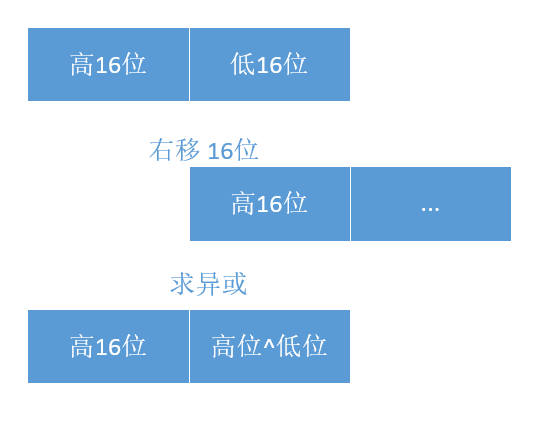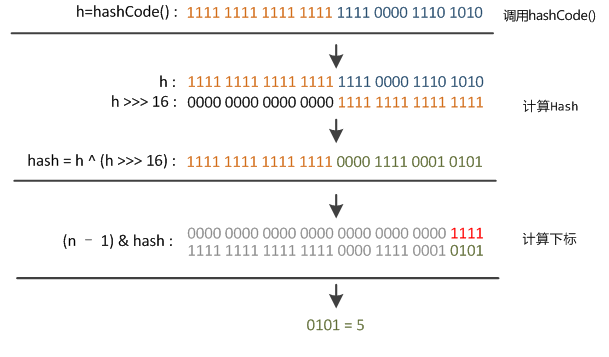（图片来自 http://yikun.github.io/2015/04/01/Java-HashMap%E5%B7%A5%E4%BD%9C%E5%8E%9F%E7%90%86%E5%8F%8A%E5%AE%9E%E7%8E%B0/

### 5.HashMap 中的初始化/扩容方法 resize() **

    //如果超出阈值，就得扩容
if (++size > threshold)
resize();


   final Node<K,V>[] resize() {
//复制一份当前的数据
Node<K,V>[] oldTab = table;
//保存旧的元素个数、阈值
int oldCap = (oldTab == null) ? 0 : oldTab.length;
int oldThr = threshold;
int newCap, newThr = 0;
if (oldCap > 0) {
if (oldCap >= MAXIMUM_CAPACITY) {
threshold = Integer.MAX_VALUE;
return oldTab;
}
//新的容量为旧的两倍
else if ((newCap = oldCap << 1) < MAXIMUM_CAPACITY &&
oldCap >= DEFAULT_INITIAL_CAPACITY)
//如果旧容量小于等于 16，新的阈值就是旧阈值的两倍
newThr = oldThr << 1; // double threshold
}
//如果旧容量为 0 ，并且旧阈值>0，说明之前创建了哈希表但没有添加元素，初始化容量等于阈值
else if (oldThr > 0) // initial capacity was placed in threshold
newCap = oldThr;
else {               // zero initial threshold signifies using defaults
//旧容量、旧阈值都是0，说明还没创建哈希表，容量为默认容量，阈值为 容量*加载因子
newCap = DEFAULT_INITIAL_CAPACITY;
newThr = (int)(DEFAULT_LOAD_FACTOR * DEFAULT_INITIAL_CAPACITY);
}
//如果新的阈值为 0 ，就得用 新容量*加载因子 重计算一次
if (newThr == 0) {
float ft = (float)newCap * loadFactor;
newThr = (newCap < MAXIMUM_CAPACITY && ft < (float)MAXIMUM_CAPACITY ?
(int)ft : Integer.MAX_VALUE);
}
//更新阈值
threshold = newThr;
//创建新链表数组，容量是原来的两倍
@SuppressWarnings({"rawtypes","unchecked"})
Node<K,V>[] newTab = (Node<K,V>[])new Node[newCap];
table = newTab;
//接下来就得遍历复制了
if (oldTab != null) {
for (int j = 0; j < oldCap; ++j) {
Node<K,V> e;
if ((e = oldTab[j]) != null) {
//旧的桶置为空
oldTab[j] = null;
//当前 桶只有一个元素，直接赋值给对应位置
if (e.next == null)
newTab[e.hash & (newCap - 1)] = e;
else if (e instanceof TreeNode)
//如果旧哈希表中这个位置的桶是树形结构，就要把新哈希表里当前桶也变成树形结构
((TreeNode<K,V>)e).split(this, newTab, j, oldCap);
else { //保留旧哈希表桶中链表的顺序
Node<K,V> loHead = null, loTail = null;
Node<K,V> hiHead = null, hiTail = null;
Node<K,V> next;
//do-while 循环赋值给新哈希表
do {
next = e.next;
if ((e.hash & oldCap) == 0) {
if (loTail == null)
loHead = e;
else
loTail.next = e;
loTail = e;
}
else {
if (hiTail == null)
hiHead = e;
else
hiTail.next = e;
hiTail = e;
}
} while ((e = next) != null);
if (loTail != null) {
loTail.next = null;
newTab[j] = loHead;
}
if (hiTail != null) {
hiTail.next = null;
newTab[j + oldCap] = hiHead;
}
}
}
}
}
return newTab;
}


• 新初始化哈希表时，容量为默认容量，阈值为 容量*加载因子
• 已有哈希表扩容时，容量、阈值均翻倍
• 如果之前这个桶的节点类型是树，需要把新哈希表里当前桶也变成树形结构
• 复制给新哈希表中需要重新索引（rehash），这里采用的计算方法是
• e.hash & (newCap - 1)，等价于 e.hash % newCap

### 6.HashMap 的获取方法 get() **

HashMap 另外一个经常使用的方法就是 get(key)，返回键对应的值:

(key == null ? k == null : key.equals(k))


public V get(Object key) {
Node<K,V> e;
//还是先计算 哈希值
return (e = getNode(hash(key), key)) == null ? null : e.value;
}

final Node<K,V> getNode(int hash, Object key) {
Node<K,V>[] tab; Node<K,V> first, e; int n; K k;
//tab 指向哈希表，n 为哈希表的长度，first 为 (n - 1) & hash 位置处的桶中的头一个节点
if ((tab = table) != null && (n = tab.length) > 0 &&
(first = tab[(n - 1) & hash]) != null) {
//如果桶里第一个元素就相等，直接返回
if (first.hash == hash &&
((k = first.key) == key || (key != null && key.equals(k))))
return first;
//否则就得慢慢遍历找
if ((e = first.next) != null) {
if (first instanceof TreeNode)
//如果是树形节点，就调用树形节点的 get 方法
return ((TreeNode<K,V>)first).getTreeNode(hash, key);
do {
//do-while 遍历链表的所有节点
if (e.hash == hash &&
((k = e.key) == key || (key != null && key.equals(k))))
return e;
} while ((e = e.next) != null);
}
}
return null;
}


• 先计算哈希值;
• 然后再用 (n - 1) & hash 计算出桶的位置;
• 在桶里的链表进行遍历查找。

## 总结

1.HashMap 有那么多优点（见文首），也有个缺点：不是同步的

Map m = Collections.synchronizedMap(new HashMap(...));


2.HashMap 三个视图返回的迭代器都是 fail-fast 的：如果在迭代时使用非迭代器方法修改了 map 的内容、结构，迭代器就会报 ConcurrentModificationException 的错。

3.当 HashMap 中有大量的元素都存放到同一个桶中时，这时候哈希表里只有一个桶，这个桶下有一条长长的链表，这个时候 HashMap 就相当于一个单链表，假如单链表有 n 个元素，遍历的时间复杂度就是 O(n)，完全失去了它的优势。

4.为什么哈希表的容量一定要是 2的整数次幂?

1. 使用减法替代取模，提升计算效率；
2. 为了使不同 hash 值发生碰撞的概率更小，尽可能促使元素在哈希表中均匀地散列。

5.HashMap 允许 key, value 为 null，同时他们都保存在第一个桶中。

public V put(K key, V value) {
//先调用 hash() 方法计算位置
return putVal(hash(key), key, value, false, true);
}


static final int hash(Object key) {
int h;
return (key == null) ? 0 : (h = key.hashCode()) ^ (h >>> 16);
}


6.HashMap 中 equals() 和 hashCode() 有什么作用？

HashMap 的添加、获取时需要通过 key 的 hashCode() 进行 hash()，然后计算下标 ( n-1 & hash)，从而获得要找的同的位置。

7.你知道 hash 的实现吗？为什么要这样实现？

## Thanks

https://docs.oracle.com/javase/8/docs/api/java/util/HashMap.html

http://blog.csdn.net/eson_15/article/details/51158865

http://blog.csdn.net/q291611265/article/details/46763053

http://yikun.github.io/2015/04/01/Java-HashMap%E5%B7%A5%E4%BD%9C%E5%8E%9F%E7%90%86%E5%8F%8A%E5%AE%9E%E7%8E%B0/

https://github.com/GeniusVJR/LearningNotes/blob/master/Part2/JavaSE/HashMap%E6%BA%90%E7%A0%81%E5%89%96%E6%9E%90.md

http://www.nowamagic.net/librarys/veda/detail/1273